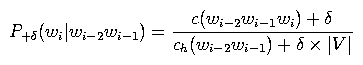# 3. Part 2: Implement “+delta” smoothing

In this part, you will write code to compute LM probabilities for a trigram model smoothed with “+delta” smoothing. This is just like “add-one” smoothing in the readings, except instead of adding one count to each trigram, we will add delta counts to each trigram for some small delta (e.g., delta=0.0001 in this lab). This is just about the simplest smoothing algorithm around, and this can actually work acceptably in some situations (though not in large-vocabulary ASR). To estimate the probability of a trigram P+delta(wi | wi-2 wi-1) with this smoothing, we takewhere |V| is the size of the vocabulary. (Note: in the above equation and the rest of the document, we abbreviate count(.) as c(.) and counthist(.) as ch(.).)

Your job in this part is to fill in the function `get_prob_plus_delta()`. This function should return the value P+delta(wi | wi-2 wi-1) given a trigram wi-2 wi-1 wi. You will be provided with the count of the trigram as well as the count of the bigram history (which you computed for Part 1), in addition to the vocabulary size. This routine corresponds to step (B) in the pseudocode listed in Section 2.1.

Your code will again be compiled into the program EvalLMLab3. To compile this program with your code, type
 ```smk EvalLMLab3 ```
To run this program on the same Switchboard training and test set used in Part 1, run
 ```lab3p2a.sh ```
The “correct” output can be found in the file p2a.out in ~stanchen/e6884/lab3/. Again, you should be able to match this output just about exactly. In this script, the program is set up to print the smoothed probability you compute as well as the trigram and bigram history count for each trigram in the evaluation data.

The instructions in lab3.txt will ask you to run the script lab3p2b.sh, which does the same thing as lab3p2a.sh except on a different test set.